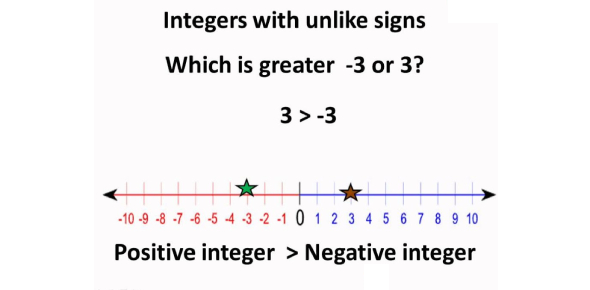# Quiz On Integers For Grade 6

Approved & Edited by ProProfs Editorial Team
At ProProfs Quizzes, our dedicated in-house team of experts takes pride in their work. With a sharp eye for detail, they meticulously review each quiz. This ensures that every quiz, taken by over 100 million users, meets our standards of accuracy, clarity, and engagement.
| Written by Kenkelmann
K
Kenkelmann
Community Contributor
Total Contribution - 2 | Total attempts - 30,782
Questions: 10 | Attempts: 28,301SettingsHello there! Check out this incredible quiz on integers that is designed for the students of grade 6th. This quiz covers mathematics topics of comparing, adding, and subtracting integers. So, if you think you're a pro at integers, then you must take this quiz to test and refresh your memory. If you score more than 70% score, it means that you've passed this quiz. So, are you ready? Let's start then!

• 1.

### Put in order from least to greatest: 12, 0, -1, -13, 4

• A.

0, -1, -13, 4, 12

• B.

12, 4, 0, -1, -13

• C.

-1, -13, 0, 4, 12

• D.

-13, -1, 0, 4, 12

D. -13, -1, 0, 4, 12
Explanation
The given numbers are arranged in ascending order from least to greatest. The correct order is -13, -1, 0, 4, 12.

Rate this question:

• 2.

### -4 + (-5) =

• A.

1

• B.

-9

• C.

-1

• D.

9

B. -9
Explanation
The given expression is -4 + (-5). When adding two negative numbers, we add their absolute values and keep the negative sign. So, the absolute values of -4 and -5 are 4 and 5 respectively, and their sum is 9. Since both numbers are negative, the sum will be negative as well. Therefore, the correct answer is -9.

Rate this question:

• 3.

### -3 + 7

• A.

-10

• B.

10

• C.

4

• D.

-4

C. 4
Explanation
The given expression -3 + 7 can be simplified by adding the two numbers together. -3 + 7 equals 4.

Rate this question:

• 4.

### -2 - (-4)

• A.

2

• B.

-2

• C.

6

• D.

-6

A. 2
Explanation
The given expression is -2 - (-4). To subtract a negative number, we change the subtraction sign to addition and change the sign of the number being subtracted. So, -2 - (-4) becomes -2 + 4. Adding -2 and 4 gives us 2. Therefore, the correct answer is 2.

Rate this question:

• 5.

### -9 - 7

• A.

-2

• B.

2

• C.

16

• D.

-16

D. -16
Explanation
The correct answer is -16 because when we subtract a negative number from another negative number, it is equivalent to adding the absolute values of the numbers and keeping the negative sign. In this case, we have -9 - 7, which is equal to -16.

Rate this question:

• 6.

### Evaluate  h - 3 for h = 1

• A.

4

• B.

2

• C.

-2

• D.

-4

C. -2
Explanation
To evaluate h - 3 for h = 1, we substitute the value of h into the expression. So, h - 3 becomes 1 - 3, which simplifies to -2. Therefore, the correct answer is -2.

Rate this question:

• 7.

### What will be the negative value of – 13?

• A.

-14

• B.

14

• C.

-12

• D.

13

D. 13
Explanation
When you negate a negative number, it becomes positive. So, if you have -13 and you want its negative value, it is simply 13.

Rate this question:

• 8.

### Write down the predecessor of – 5.

• A.

-4

• B.

-6

• C.

5

• D.

6

B. -6
Explanation
The predecessor of -5 is the number that comes before it in the number line. In this case, the number that comes before -5 is -6.

Rate this question:

• 9.

### Which of these integers will come between the following pair of integers? – 8 and – 13

• A.

9, -10, -11, -12

• B.

-12, -11, -10, -9

• C.

12, 11, 10, 9

• D.

None of the above

B. -12, -11, -10, -9
Explanation
The integers that come between -8 and -13 are -12, -11, -10, and -9. Other options contain positive integers which could not exist between -8 and -13.

Rate this question:

• 10.

### What will be the sum of this integer equation? (- 8) + (+ 5) + (- 3) + (- 2)

• A.

-5

• B.

-7

• C.

-8

• D.

8Back to top